## 表征材料行为## 动力力学分析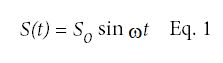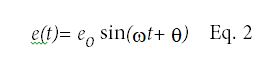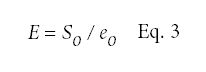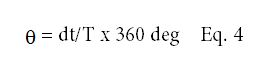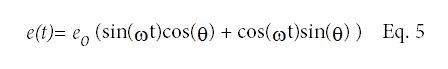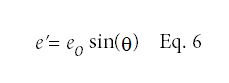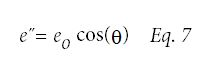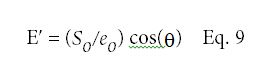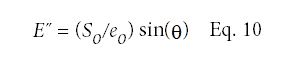Dt = 0.012秒

T = 0.1秒(10hz)

So = (12.521 - 4.779)/2 = 3.871 Mpa

eo= (1.0244-0.6049)/(2 × 100) = 0.00209 mm/mm

Q = 0.012/0.1 x 360 = 43.2度

Eʹ= 3.871/0.00209 x cos (43.2) = 1,350 Mpa

E“= 3.871/0.00209 × sin(43.2) = 1268兆帕

ASTM D638塑料拉伸性能的标准试验方法规定了聚丙烯杨氏模量的测定。图6为拉伸至破坏的聚丙烯试样的应力-应变图。E = 1884 MPa